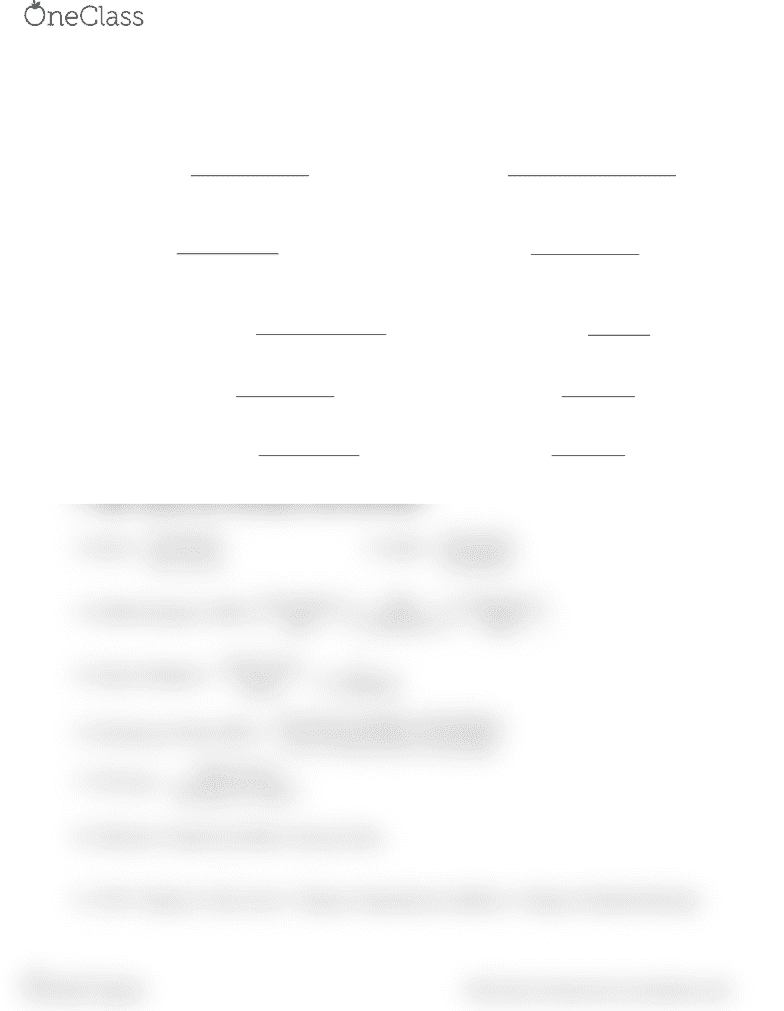Textbook Notes (280,000)
US (110,000)
NU (1,000)
FINA (60)
Chapter 18

# FINA 2201 Chapter Notes - Chapter 18: Retained Earnings, Net Income

Department
Finance & Insurance
Course Code
FINA 2201
Professor
Ma Linlin
Chapter
18

This preview shows half of the first page. to view the full 2 pages of the document.Formula Sheet
FINA 2201
(Note: This sheet does not list many formulas that are important for the exam)
1. Current Ratio =
sLiabilitieCurrent
AssetsCurrent
2. Quick Ratio =
sLiabilitieCurrent
InventoryAssetsCurrent
3. Debt Ratio =
Total Liabilities
Total Assets
4. Times Interest Earned =
OperatingIncome
Interest Expense
5. Days Sales Outstanding =
365/
Sales
ReceivableAccounts
6. Inventory Turnover Ratio =
Inventory
Sales
7. Fixed Asset Turnover=
setsNetFixedAs
Sales
8. Total Asset Turnover=
sTotalAsset
Sales
9. Operating Profit Margin =
OperatingProfit
Sales
10. Net Profit Margin =
11. Basic Earning Power=Operating Profits/Total Assets
12. ROA =
AssetsTotal
IncomeNet
13. ROE =
14. DuPont Equation: ROE=
Net Income Sales TotalAssets
Sales TotalAssets Equity

 


 
  
15. Equity Multiplier =
1
1
Total Assets
Equity DebtRatio
16. Earnings per Share (EPS) =
17. P/E ratio =
SharePer Earnings
Price Market
18. M/B ratio= Market price/Book value per share
19. AFN= Change in Total Assets - Change in Spontaneous Liabilities - Change in Retained Earnings
goutstandin sharescommon of No.
ers shareholdto available income Net
find more resources at oneclass.com
find more resources at oneclass.com